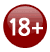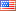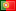& get our free guide
Starting Hands Revealed

We hate spam too!
We value your privacy and never share your email. All our emails have an opt-out link. Click it, and you will never hear from us again.

# Hold'em Statistics Basics

##### Essentials

In Texas Hold'em poker and in gambling in general, what matter the most is the probability of an event. If you throw two dice, what is the probability that they sum up to 8? In you go to the race track, what is the probability that "Proud Spell" finishes in the top three. Similarly, if you play no-limit full ring holdem and hold rockets, what is the probability that your pair of aces get cracked?

Understanding such ideas does not require a Ph.D. in Statistics. Most of the concepts related to holdem odds are just a question of counting. Yes, counting, like counting scenarios. As there are 52 cards in the deck, there are only a finite number of scenarios in each case. C(n,k) is one of the cornerstone for counting. Of course when we say counting we do not mean counting cards like in black jack, we mean that in order to calculate probabilities, it is necessary to first count all the possible corresponding scenarios.

##### C(n,k), n choose k

This very useful function tells us how many ways there are to pick a subset of k elements from a larger set of n elements. For example if you play lotto and must choose 6 numbers from 1 to 55, the number of ways to do that is C(55,6), because we have to pick 6 numbers among 55. It turns out that C(55,6)≈29 millions, so good luck to you. The mathematical formula is C(n,k)=n!/{(n-k)!k!}, where x! stands for x factorial, ie x*(x-1)*(x-2)...*2*1. If you are not confortable wirth math, do not get scared! Usually we will only deal with very simple case of this formula, and anyway there are short-cuts which provide very easy to remember rules of thumbs.

##### Number of starting hands

Let us see how useful this formula can be. In holdem we are first dealt two hole cards. How many possible hole cards can we be dealt?
Picking the two hole cards is like randomly choosing two cards among the 52 from the deck, so the answer is C(52,2)=52*51/2 = 1326 ways. Note that in this calculation, no distinction is made between AA and AA♠. We will see in Starting Hands Odds how to remedy this.

##### Number of possible hands with four players

The previous example was simple, let us see a more complicated one, just for exposition's sake. Four poker players play texas holdem. What is the total number of hands that can occur?
- As before, we have to assign 2 cards to the first player, the number of ways to do that is C(52,2)=1326,
- Then we must deal two cards to the second player, as 50 cards are left in the deck, it is C(50,2)=1225,
- Same for the last two players, C(48,2)=1128 and C(46,2)=1035.
- Finally in order to make the board, we pick 5 cards from the remaining 44 in the deck, i.e. c(44,5)≈1 million.
The total number of hands is the product of all counts, that is ≈ 2E+18, or 1 billion of billion cases. This seems like an astronomical number, and it truly is. But practically a lot of the situations are similar to each other, so there is no need to investigate that many cases.

 BEST POKER ROOMSPokerStarsCarbon Poker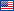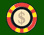PartyPokerTitan Poker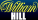William Hill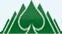Everest Poker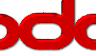Bodog PokerCake Poker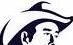DoylesRoomFull Tilt